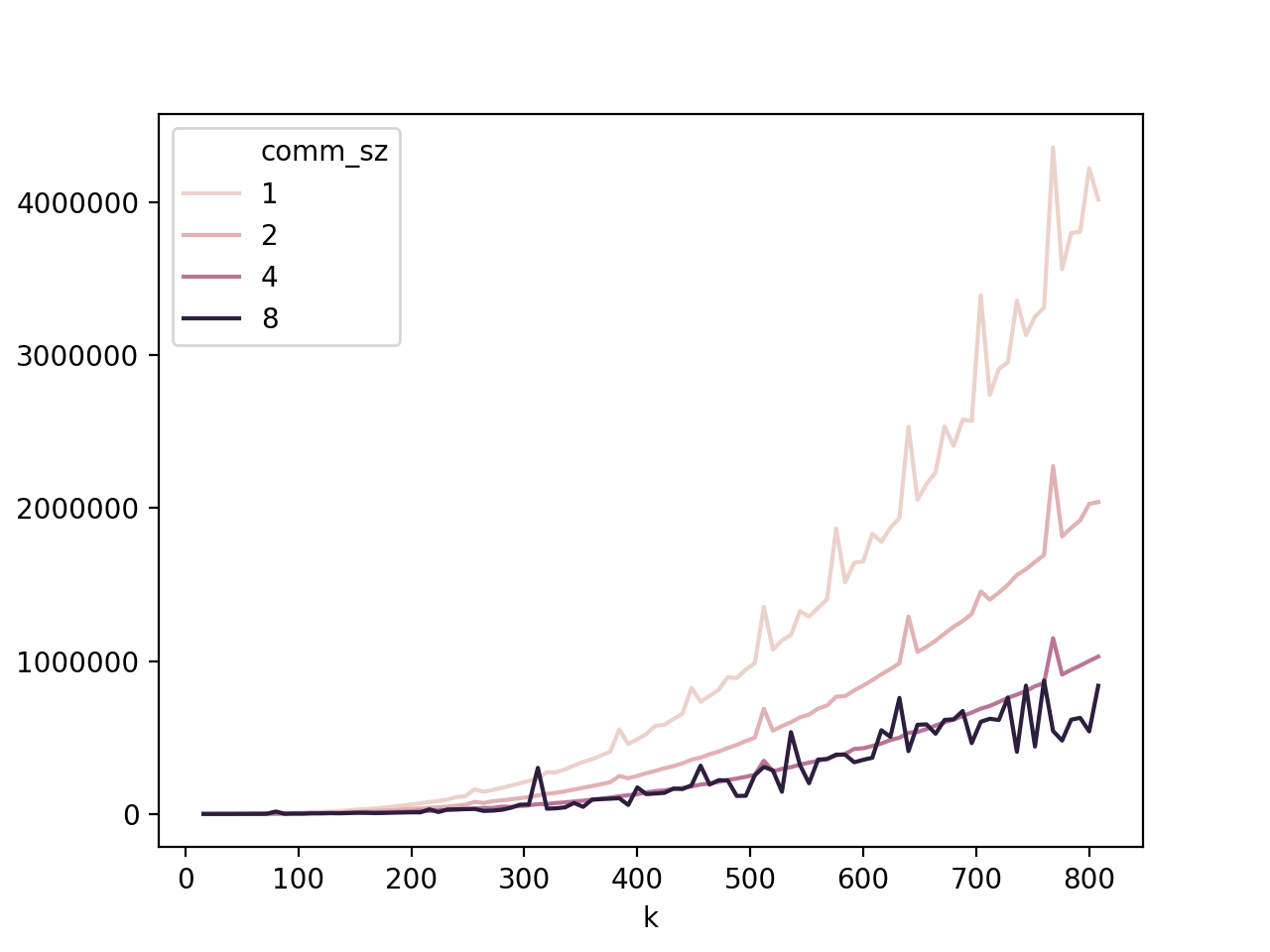# Matrix Multiplication

This was a project comparing performance of matrix-matrix multiplication with different sizes of matrices and different amounts of processes, using MPI.

import pandas as pd
import seaborn as sns


## Parallelization Methodology (PCAM)

### Partitioning scheme of tasks and data required to compute C

Matrix multiplication is as follows: Let A•B = C, where \begin{equation} c{m,n} = \sum{i=0}^{k-1} a{m,i} • b{i,n} \end{equation} where m,n are the row/column of the cell c, and k is the length of the inner dimension of A•B.

Thus, we can see that there is an intrinsic independance between many portions of the computation, and thus can be rather easily parallelized. For each cell of the matrix, we can divide the computation sum-wise, meaning each component of the sum of each cell would be a separate task. The first terms of every sum could be assigned to the first “worker”, the 2nd to the 2nd “worker”, etc..

### Communication required to partition A and B

To distribute the task of multiplying the two matrices together, one must first make assumptions: 1. There will never be more processes than matrix rows 2. All matrix dimensions divide evenly into the number of processes

This allows us to assume that the array can be split evenly and distributed to the workers without considering the chance for uneven distribution, or having idle workers with no portion of the matrix to work on.

The tasks can divided by calculating the size of the inner dimension of A•B, and then distributing n rows of B and columns of A per worker, where n = k / num_workers.

Once each portion of A and B have been ditributed, recall that the assumption made was that the dimensions of A and B are multiples of the number of processes. This means that although ideally we would have enough workers to map a worker to every term in the summation (first terms to first worker, second terms to second worker, etc.), realistically a matrix could be size 1024x1024, whereas your CPU might only have 4 to 8 cores (latest i7 CPUs have around 8 cores, 16 threads).

Thus, each worker would perform numerous steps: not only would they multiply the cells of A and B together, but they would also partially complete the summation as well, based on how many rows / columns each worker is responsible for (the more rows / columns versus number of workers, the more terms each worker has to compute and then sum together).

Finally, when each worker has successfully completed their portion of the multiplication, all individual results are summed together using MPI_Reduce along with the MPI operator MPI_Sum and the result is placed into C. The result of this step is that C represents the final matrix multiplication of A•B.

## Tables

The output below is the data used for the tables:

df

comm_szkmntime
01323232244
116464643021
2112812812816256
31256256256165050
42323232155
526464641050
621281281288044
7225625625678905
84323232229
94646464639
1041281281284742
11425625625633623
128323232174
138646464422
1481281281283973
15825625625632972

### Timing

comm_sz32x3264x64128x128256x256
1244302116256165050
21551050804478905
4229639474233623
8174422397332972

### Speedup

comm_sz32x3264x64128x128256x256
11111
21.562.882.022.09
41.064.723.424.90
81.47.154.095.00

### Efficiency

comm_sz32x3264x64128x128256x256
11111
20.781.41.011.05
40.271.180.861.23
80.160.890.510.63

## Graph of fine-grain output, for matrices of size 8n, cores = {1, 2, 4, 8}

%matplotlib notebook

<IPython.core.display.Javascript object><matplotlib.axes._subplots.AxesSubplot at 0x126afcad0>


## Analysis of Results

### Timing

We can see from both the graph and the timing results that as the number of cores increases the rate at which the timing increases is more gradual. In most cases, what is more important than the time itself is the efficiency at which the algorithm scales with respect to the size of the input, and so we can see that having more cores is quite beneficial to the speed of this algorithm.

### Speedup

for the smaller 32x32 and 64x64 examples there is a noticeable improvement in speed going from 1 to 2 cores, and again from 4 to 8 cores. While smaller input sizes generally lead to more erratic results, we can see from the graph that the relative timings of 4 and 8 cores (especially at higher matrix sizes) is quite similar, which might be due to the fact that the server which this was run on might not actually have 8 cores, or may be busy with other tasks from other users at different times. Cache size, context switching, memory bandwidth / timings, all still have an impact on the performance of parallelism which is made evident by the rather erratice nature of the graph above.

We can also see that although the speed does increase relative to the number of cores, the speeds tend to approach a fixed value, which suggest that there is a diminishing return of speedup the more cores are added (amdahl’s law). This does not mean that parallelism is not worthwhile, however we should remain aware of its limitations, and its dependency on your implementation (see Strassen’s matrix multiplication algorithm, which is a faster yet more complex implementation).

### Efficiency

With the exception of the 4-core 256x256 example in the table above, we can also see the efficiency per core is better the fewer cores there are which makes sense as although the time to compute might take longer, there is less overhead of actually sending the computations off to other cores, and this overhead increases the more cores there are.

There is also an increase in efficiency as the size of the matrix increases, since the more work you can assign each core, the more efficient the computation becomes.